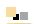# void star games

* play * code * learn *

##Flash ActionScript Mines Tutorial 2 BackNext

Tiles Class

Now we can start coding. Let's create a class handling a tile. From the File menu, select New, then ActionScript file. Save it in the same forlder, under the name "Tile.as". Copy the following text into this file and save it:

package {
import flash.display.BitmapData;
import flash.display.Sprite;
import flash.display.Stage;
import flash.utils.Timer;
import flash.geom.Matrix;
import flash.events.MouseEvent;
import Math;

public class Tile {
}
}

The remaining of the code will go inside the pair of curly braces following the class name. First, we need to add some attributed that will store a version of each of the 3 tiles that we created in the stage for each tile. Add the following line inside the class definition, right at the top:

var closedClip, highlightedClip, flaggedClip, explodedClip, bombClip;

Then we need an attribute to tell us which of these states the tile is in, then another one to store the parent of the tile, which is the stage, since we need to reference it, and one to tell us if there is a bomb in that place or not. We make this attribute public because we might need easy access to it from outside of the object. We need a couple of attributes to keep track of the table coordinates of the tile and of its coordinates on the stage (pixels). Last, we would like to keep track of its size, so that we can reprogram the game easily with a different size for the tiles. We can assume that they are squares. So add the following list of attributes right under the first ones:

var parent, table;
var x, y, col, row;
var orgx=70, orgy=70, size;
public var state, isBomb;

Now add the following function that will take an instance of one of the clips that we created before and converted to symbols and position it within the tile object at the stage coordinates stored for the tile.

public function positionClip(clip) { clip.x = x; clip.y = y; }

Let us now write a function that initializes a tile. Add the following function above the previous one but below the declarations of attributes:

public function Tile(theStage, c, r, tab, tileSize=30) {
col = c; row = r; size = tileSize;
x = orgx+r*size; y = orgy+c*size;
parent = theStage;
table = tab;
state = 0;
isBomb = false;
closedClip = new TileClosed();
positionClip(closedClip);
}

and the replicate the last 3 lines in the function for each of the clip attributes we declared above, using their respective symbol class name that we entered for them when we converted the drawings to symbols.

Now we are ready to create a tile and add it to the screen. Go back to the fla file and open a window called Actions from the Window menu. Make sure that Layer 1: Frame 1 is selected in the left bottom area of the window. Then add the following two lines to it:

import Tile;
var t = new Tile(this.stage, 1,1, null);

Save all the files and then test the program with Ctrl-Enter . You should see the stage such as you've set it up, plus a tile on the top left but not quite in the corner. First, we need to hide all the clips but the closed one. For this, add the following line to the initialization function:

highlightedClip.visible = false;
and then add a similar line for all the others.

Go back to the fla file mines and open the Actions window. Let's add all the tiles now to the table. Replace the line declaring the variable t with the following:

var t; for (var i=0; i & lt; 9; i++) {
for (var j=0; j < 9; j++) {
t = new Tile(this.stage, i, j, null);
}
}

Now run the program again. If everything went fine, you should see all the tiles in the tables, perfectly lines up with the background square.

Before we move forward, let us add a couple of functions to the class Tile that will be useful later.

public function setBomb() {
isBomb = true;
}
public function setState(s:int) {
state = s;
closedClip.visible = false;
highlightedClip.visible = false;
flaggedClip.visible = false;
explodedClip.visible = false;
bombClip.visible = false;
switch (state) {
case 0:
closedClip.visible = true;
break;
}
}

then complete the second function with 4 other cases for each of the clips. Remove all the instructions from the initialization function that make the clips visible/invisible, and replace them by a single call to the function setState with the parameter being 0.

Before we move forward, we can remark that a parameter of 0 will not be very informative for the reader of the code, and we would have to go back to this function and remember what each of these states is. What we can do instead, is to declare some new attributes that are named after the states in which the tile can be, and use those instead of the numbers. We can make them public because we might need them from outside the class too.

So let’s add the following to the list of attributes:

public var closedSt=0, highlightedSt = 1, flaggedSt = 2, explodedSt = 3, bombSt = 4, emptySt = 5;